# 20200611上证指数分析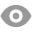161 |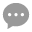6 |桥博士助教 | 2020-6-11 15:08:10 | 显示全部楼层 |阅读模式
 20200611上证指数 请用量化的思维来描述一下上证指数6月11日的走势和形态 并且分析出明天的涨跌概率

2020-6-11K线测试结果如下：
 天数 胜率 盈亏比 胜率盈亏 交易次数 C在N日均线上方 1 54.27% 1.06 0.12 164 20 2 54.78% 1.14 0.17 157 3 49.01% 1.2 0.08 151 1 52.94% 1.1 0.11 153 30 2 57.14% 1.16 0.23 147 3 57.14% 1.34 0.34 140 1 52.05% 1.14 0.11 171 60 2 51.23% 1.31 0.18 162 3 48.34% 1.29 0.11 151

FUND:=10000000;
LOTS:=INTPART(FUND*0.8/(CLOSE+FEE));//计算手数，使每次开仓金额都是800W
TR:=MAX(MAX((HIGH-LOW),ABS(REF(CLOSE,1)-HIGH)),ABS(REF(CLOSE,1)-LOW));//真实波幅
ATR:=MA(TR,26); //求26个周期内真实波幅的简单移动平均

GPJ:=O<REF(O,1);//开盘价大于前一天开盘价
SPJ:=C<REF(C,1);//收盘价大于前一天收盘价
XYX:=MIN(O,C)-L<0.35*ATR;//下影线长度0.305
SYX:=H-MAX(O,C)<0.5*ATR;//上影线长度0.451
ST:=ABS(O-C)<0.7*ATR;//ST长度0.663
KXCD:=(H-L)<1.5*ATR ;//K线长度1.42
SZQS:=C>MA(C,60);//定义上涨趋势
BT:=GPJ && SPJ && XYX && SYX && ST && KXCD && SZQS;
BT,BK(LOTS);//与前一天收盘价比高开，以收盘价买入
BARSBK=1,SP(BKVOL);//第二天，以收盘价卖出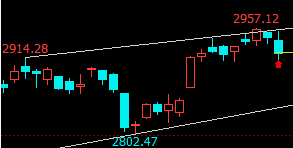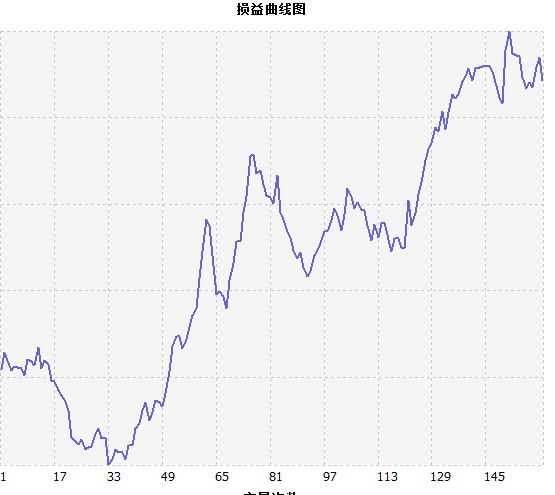今日k线测试结果为：胜率45.45%，盈亏比0.67，交易次数22.胜率盈亏--0.24.。代码如下： //定义仓位 FUND:=10000000; LOTS:=INTPART(FUND*0.8/(CLOSE+FEE));//计算手数，使每次开仓金额都是800W //定义K线 KXST:=ABS(O-C);//K线实体长度 YXCD:=H-L;//K线影线长度 BT:=KXST>YXCD*0.5;//棒体实体大于影线的0.5倍 TR : MAX(MAX((HIGH-LOW),ABS(REF(CLOSE,1)-HIGH)),ABS(REF(CLOSE,1)-LOW));//求最高价减去最低价，一个周期前的收盘价减去最高价的绝对值，一个周期前的收盘价减去最低价的绝对值，这三个值中的最大值 ATR : MA(TR,26),COLORYELLOW;//求N个周期内的TR的简单移动平均 XYX:=MIN(O,C)-L; SYX:=H-MAX(O,C); BT1:=KXST>YXCD*0.5;//棒体实体 BT2:= KXSTXYX*5 && SYX>KXST ;//倒锤线 BT4:=XYX>SYX*5 && XYX>KXST;//锤头线 //定义K线形态 KFP1:= REF(BT1,2)  ;//第一根为棒体 KFP2:=  REF(BT4,1)  ;//第二根锤头线 KFP3:= BT2 AND CHV(H,55); KFP:=KFP1 AND KFP2 AND KFP3 AND KFP4 ; KFP,BK(LOTS);//，以收盘价买入 BARSBK=1,SP(BKVOL);//第二天，卖出       当测试两根k线时，数据是，57.45%，0.7，,47次。胜率盈亏--0.023，中性偏空。         在上述情形下，当C,

 明天看涨

 今天我支持辛老师的分析

 FUND:=1000000; LOTS:=INTPART(FUND*0.8/(CLOSE+FEE));//计算手数，使每次开仓金额都是80W TR:=MAX(MAX((HIGH-LOW),ABS(REF(CLOSE,1)-HIGH)),ABS(REF(CLOSE,1)-LOW));//真实波幅 ATR:=MA(TR,26); //求26个周期内真实波幅的简单移动平均 KXST:=ABS(O-C);//K线实体长度 KXCD:=H-L;//K线长度 SYX:=H-MAX(O,C);//上影线长度 XYX:=MIN(O,C)-L;//下影线长度 DFP1:=REF(ISUP,2); //第二根为阳棒体 DFP3:=REF(ISDOWN,1); //第一根为阴棒体 DFP2:=ISDOWN AND MAX(O,C) < MIN(REF(O,1),REF(C,1));//低开低走 DFP6:= KXCD>ATR ; DFP4:=REF(C,2)>HV(REF(C,2),5);//收盘价突破形态最高价 SZQS:=C>MA(C,60);//定义上涨趋势 DFP:= DFP1  AND DFP3 AND DFP6  AND DFP2  AND DFP4 AND SZQS ; DFP,BK(LOTS);//一阳二阴，买入 BARSBK=1,SP(BKVOL);//第二天，卖出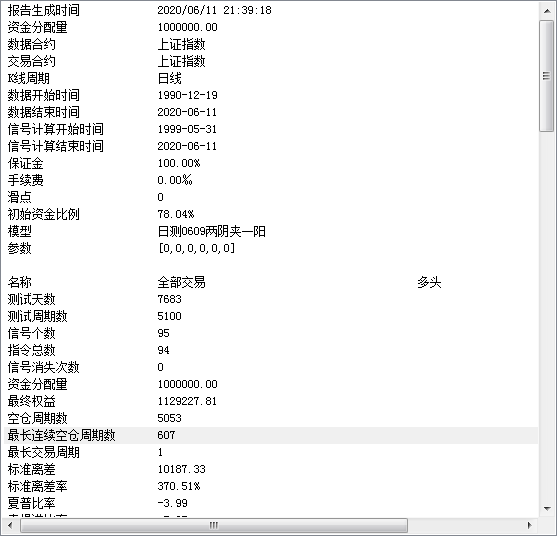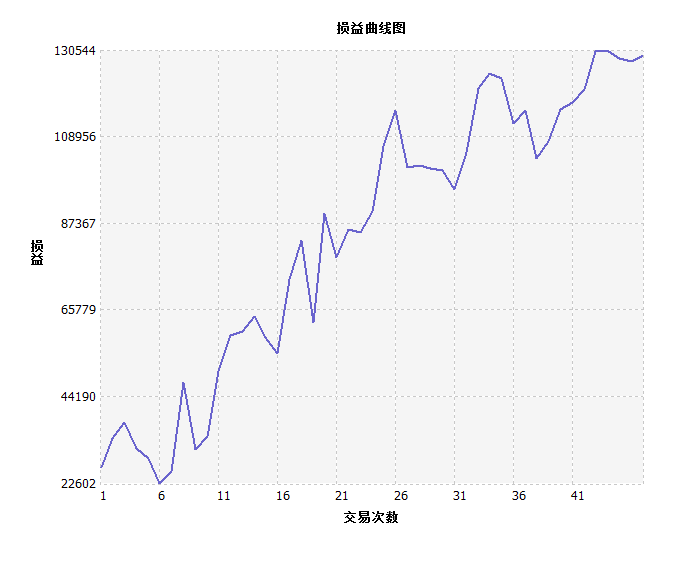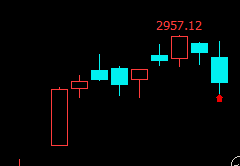胜率61.7%盈亏比1.28模型得分56胜率盈亏0.406  涨

 明天看跌

 您需要登录后才可以回帖 登录 | 立即注册 本版积分规则 回帖后跳转到最后一页166主题 1423积分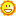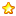1423